# Position and Speed Estimation of PMSM Based on Extended Kalman Filter Tuned by Biogeography-Based-Optimization

Position and Speed Estimation of PMSM Based on Extended Kalman Filter Tuned by Biogeography-Based-Optimization
Electronics Department, Faculty of Technology, Batna2 University, Fesdis, Batna 05078, Algeria

Electrical Engineering Laboratory, Faculty of Technology, M’Sila University, B.P 166, Ichebelia, M’Sila 28000, Algeria

Laboratoire d'Automatique Avancée et d'Analyse des Systèmes (LAAAS), Batna 2 University, Batna 05078, Alegria

Laboratoire Systèmes Electromécaniques (LSELM), Badji-Mokhtar Annaba University, Annaba 23005, Alegria

Corresponding Author Email:
samia.allaoui@univ-batna2.dz
Page:
559-568
|
DOI:
https://doi.org/10.18280/jesa.540405
13 July 2020
|
Accepted:
10 August 2021
|
Published:
31 August 2021
| Citation

OPEN ACCESS

Abstract:

In a sensorless control of PMSM based on Extended Kalman Filter (EKF), the correct selection of system and measurement noise covariance has a great influence on the estimation performances of the filter. In fact, it is extremely difficult to find their optimal values by trial and error method. Therefore, the main contribution of this work is to prove the efficiency of Biogeography-Based-Optimization (BBO) technique to obtain the optimal noise covariance matrices Q and R. The BBO and EKF combination gives a BBO-EKF algorithm, which allows to estimate all the state variables of PMSM drive particularly, the rotor position and speed. In this paper, three evolutionary algorithms namely Particle Swarm Optimization (PSO), genetic algorithms (GAs) and BBO are used to get the best Q and R of EKF. Simulations tests performed in Matlab Simulink environment show excellent performance of BBO-EKF compared to GAs-EKF and PSO-EKF approaches either in resolution or in convergence speed.

Keywords:

biogeography based optimization, extended Kalman filter, permanent magnet synchronous motors, sensorless control, state estimation

1. Introduction

In nowadays permanent Magnet Synchronous Motors (PMSMs) are widely applied in a various electromechanical system, computerized numerical control (CNC) machine tools and industrial robots because of their numerous advantages such as high-power factor, high torque to inertia ratio and high efficiency [1-3].

In most drive systems, the use of speed and position sensors in the control of PMSM seems to be a major drawback in control systems due to cost, weight and reduced reliability or even overall system dynamic performance [4-8]. Therefore, an observer is required in order to estimate the system state. This observer can efficiently replace transducers and encoders.

Currently, different control algorithms were presented in several works to eliminate mechanical sensors placed on the motor shaft [5-7]. In fact, a PMSM sensorless performance control depends mostly on the quality of the state variables and disturbances estimation.

This remains a major problem because of errors in measurement, model parameters variations, mathematical models uncertainties, and the instability when approaching zero speed range. Thus, an important effort is required to enhance quality and accuracy of the states and parameters estimation [3, 8]. For linear cases, Luenberger  and Kalman filter [10, 11] are considered as appropriate observers. However, these methods are not applicable for non-linear estimation systems. In order to overcome this drawback, several observers were presented in the previously reported works [12-18].

During the last few years, Extended Kalman Filter (EKF) was employed to estimate PMSM rotor speed and position considered as one of the best tools of state estimation using stator currents and voltages [5, 19]. Therefore, the most important benefit of the EKF over is its capability of tracking non-linear system dynamics with higher accuracy, high dynamic performance, disturbance resistance and it can operate under stochastic uncertainties (model uncertainties and inherent nonlinearities). However, the estimation performance of EKF is highly affected by system parameters values and covariance matrices Q and R of state and measurement noises, respectively . Regarding Kalman filter theory, Q and R are obtained by considering the stochastic properties of equivalent noises ; but, more often these are unknown, in most cases, the covariance matrices are employed as free tuning parameters. These matrices were first adjusted manually by trial and error methods but it was find that this procedure is ineffective because of time duration [20-22].

In order to solve this issue and prevent test computations complication, many researchers try to find a convenient way to determine the noise covariance matrix of EKF by using some intelligent methods. Genetic algorithm (GA) is proposed in Ref.  to optimizes Q and R matrices automatically. The authors  have applied an evolutionary algorithm inspired by social interactions called Particle Swarm Optimization (PSO) technique to calculate Q and R matrices optimal values.

In order to fulfill the objective of finding an efficient, simple and easy tool for PMSM position and speed estimation, the present paper proposes a new alternative intelligent technique called Biogeography-Based-Optimization (BBO) [23, 24]. As this technique is not previously widely investigated and applied for PMSM position and speed estimation, the present work is exclusively reserved for this algorithm to optimize Q and R matrices.

The principal idea of this paper is the estimation of all the state variables of PMSM drive using an EKF tuned by BBO algorithm. The proposed strategy will be performed in two steps. First, the covariance matrices Q and R are optimized off-line by the BBO algorithm, after that, these optimal values obtained are introduced into an EKF estimator that works online.

Simulation tests were carried out to investigate the effectiveness of the proposed approach and also a comparative study between BBO with both evolutionary algorithms GAs and PSO were performed.

The structure of this paper is as follows: Section 2 reviews the principle and algorithm of EKF, describes the ideas and concepts of BBO algorithm and presents the discrete PMSM model. In section 3, the proposed structure of the BBO-EKF approach used to optimize the noise covariance matrices is presented. Simulation and results are given in section 4. Finally, conclusions are drawn in section 5.

2. Theoretical Developments

2.1 Extended Kalman filter (EKF)

An EKF is an optimal recursive state estimator that can be used to simultaneously estimate states and parameters of nonlinear stochastic dynamic systems [2, 5]. This research aims to obtain the best linear estimate of the PMSM state vector according to the nonlinear dynamic equation in discrete time, as expressed below.

\left\{ \begin{align} & {{x}_{k+1}}=f({{x}_{k}},\,{{u}_{k}},\,{{w}_{k}}) \\ & {{z}_{k}}=h({{x}_{k}},\,\,{{v}_{k}}) \\ \end{align} \right.                     (1)

where, f(.) is the nonlinear function of xk, h(.) is the nonlinear function relates the state xk and the measurement zk, uk is the input vector, vk and wk represent the system and measurement noises with covariance matrices $Q=E\left[w_{k} w_{k}^{T}\right]$ and $R=E\left[v_{k} v_{k}^{T}\right]$, respectively.

Employing the first order Taylor approximation close to the reference point ($\hat{x}_{k}, \widehat{w}_{k}=0, \hat{v}_{k}=0$), the approximated linear model can be expressed as follows:

$\left\{\begin{array}{l}x_{k+1} \approx f\left(\hat{x}_{k}, u_{k}, 0\right)+F_{k}\left(x_{k}-\hat{x}_{k}\right)+W_{k}\left(w_{k}-0\right) \\ z_{k} \approx h\left(\hat{x}_{k}, 0\right)+H_{k}\left(x_{k}-\hat{x}_{k}\right)+V_{k}\left(v_{k}-0\right)\end{array}\right.$                (2)

Fk, Wk, Hk and Vk are the Jacobians determined by the following expression:

$F_{k}=\left.\frac{\partial f(x, 0)}{\partial x}\right|_{x=\hat{x}_{k}}, W_{k}=\left.\frac{\partial f\left(\hat{x}_{k}, w\right)}{\partial w}\right|_{w=0}$,

$H_{k}=\left.\frac{\partial h(x, 0)}{\partial x}\right|_{x=\hat{x}_{k}}, V_{k}=\left.\frac{\partial h\left(\hat{x}_{k}, v\right)}{\partial v}\right|_{v=0}$                  (3)

Consequently, the set of Kalman filter equations can be expressed by the recursive equations below:

$\hat{x}_{k+1 / k}=f\left(\hat{x}_{k / k}, u_{k}, 0\right)$

$P_{k+1 / k}=F_{k} P_{k / k} F_{k}^{T}+W_{k} Q W_{k}^{T}$

$K_{k}=P_{k+1 / k} H_{k}^{T}\left(H_{k} P_{k+1 / k} H_{k}^{T}+V_{k} R V_{k}^{T}\right)^{-1}$

$\hat{x}_{k+1 / k+1}=\hat{x}_{k+1 / k}+K_{k}\left(z_{k}-h\left(\hat{x}_{k+1 / k}, 0\right)\right)$

$P_{k+1 / k+1}=P_{k+1 / k}-K_{k} H_{k} P_{k+1 / k}$           (4)

where, $\hat{x}_{k+1 / k}$ is the predicted state vector at the $(k+1) t h$ instant, $\hat{x}_{k+1 / k+1}$ is the corrected estimation vector, $P_{k+1 / k}$ is the error covariance matrix at the $(k+1) t h$ instant, $P_{k+1 / k+1}$ is the corrected error covariance matrix and $K_{k}$ is the Kalman filter gain. The structure of the EKF is illustrated in Figure 1.

## 1.png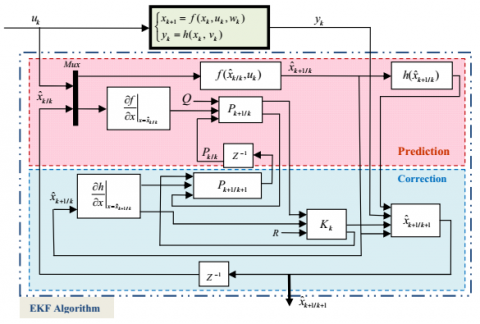Figure 1. Extended Kalman filter structure

2.2 Biogeography based optimization (BBO)

As an evolutionary algorithm (EA), BBO consists in studying the distribution of biological species through time and space between islands in search of more convivial habitats. Dan Simon was the first to introduce this algorithm in 2008 for setting optimization problems .

In this algorithm, the population corresponds to a number of islands (habitats), each possible solution is considered as an island. The characteristics that describe the habitability are called the habitat suitability index (HSI) employed to measure the solution quality which is equivalent to the fitness function in other EA like AGs and PSO. The effectiveness of each solution that characterizes habitability named suitability index variables (SIV) [25-27].

Islands indicate solutions, thus a solution with a high HSI will be able to export its dominant genes to adapt the other islands. In contrast solutions with low HIS, will be able to receive the dominant genes to be adapted.

In BBO, each habitat has its own rate of immigration λj and its rate of emigration µj. These two rates are based on the number of species in the habitat. In Figure 3, E and I illustrate the highest rates of immigration and emigration, respectively, and S0 denotes species equilibrium number and Smax is the maximum number of species [24-27].

$\lambda_{j}=I\left(1-\frac{S}{S_{\max }}\right)$             (5)

$\mu_{j}=E \frac{S}{S_{\max }}$          (6)

where, λj and μj are respectively the immigration and the emigration rates for the jth habitat. S is the species number in the jth habitat; BBO migration is employed to modify both the existing solution and the island. The probability of choosing the jth habitat as the emigration the probability of selecting jth habitat as emigrating habitat is computed as follows:

$P_{j}=\frac{\mu_{j}}{\sum_{j=1}^{N P} \mu_{j}}$, for $\mathrm{j}=1,2, \ldots, \mathrm{NP}$             (7)

Figure 2(a) shows the details of the migration procedure (i.e. how to select emigrating habitat) in the BBO algorithm.

where, NP denotes population size, rand(0;1) is a uniformly distributed random number in the interval [0, 1] and Hi(j) is the jth SIV of the solution Hi.

However, the mutation is employed to rise population diversity to obtain the right solutions. Operator mutation shown in Figure 2(b), randomly modifies the SIV of a habitat according to the mutation rate [25-27].

The mutation rate mj for the jth habitat with j species number is expressed as follows:

$m_{j}=m_{\max }\left(\frac{1-P_{j}}{P_{\max }}\right)$           (8)

where, mmax denotes the maximum mutation rate; Pmax represents the maximum species count probability and Pj is jth habitat species count probability.

## 2.png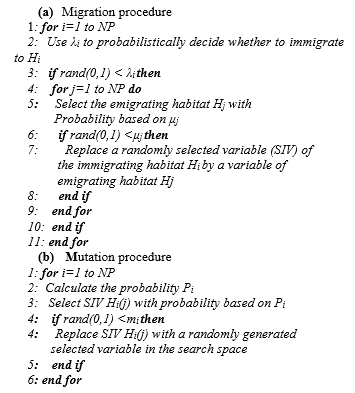Figure 2. Migration and mutation of BBO algorithm

## 3.png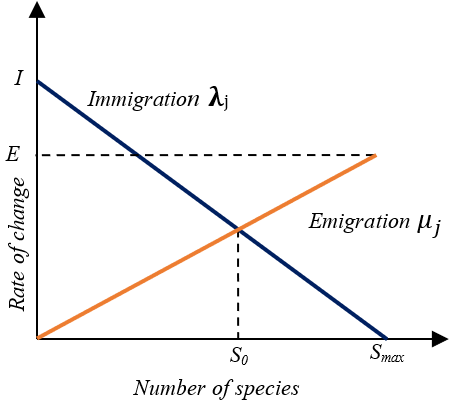Figure 3. Emigration and immigration rates species model

Therefore, in Figure 4, are illustrated the main steps of the standard BBO algorithm.

## 4.png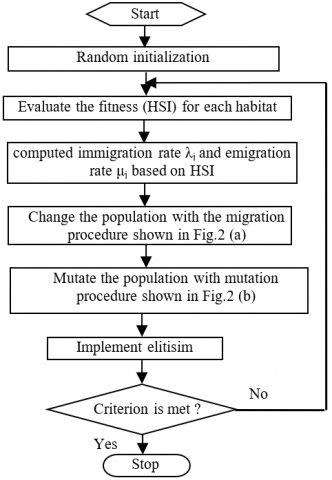Figure 4. Standard BBO algorithm flowchart

2.3 Mathematical model of PMSM

The PMSM model can be expressed in the synchronous reference frame (d, q), fixed to the rotor by considering four state variables, the stator currents (ids, iqs) and the mechanical variables (rotor speed ωr and position θr), the stator voltages (vds, vqs) sush as control variables, the measurements y, state and measurement noises (w and v) are defined respectively, as follows:

$x(t)=\left[\begin{array}{llll}x_{1} & x_{2} & x_{3} & x_{4}\end{array}\right]^{T}=\left[\begin{array}{llll}i_{d s} & i_{q s} & \omega_{r} & \theta_{r}\end{array}\right]^{T}$       (9)

$u(t)=\left[\begin{array}{ll}v_{d s} & v_{q s}\end{array}\right]^{T}$           (10)

$z=\left[\begin{array}{ll}i_{d s} & i_{q s}\end{array}\right]^{T}$             (11)

$w=\left[\begin{array}{llll}w_{1} & w_{2} & w_{3} & w_{4}\end{array}\right]^{T}$                 (12)

$v=\left[\begin{array}{ll}v_{1} & v_{2}\end{array}\right]^{T}$            (13)

Then, the PMSM model is expressed by the continuous nonlinear representation below:

$f(x, u, w)=\left[\begin{array}{c}\dot{x}_{1} \\ \dot{x}_{2} \\ \dot{x}_{3} \\ \dot{x}_{4}\end{array}\right]= \left[\begin{array}{c}-\frac{R_{s}}{L_{d}} x_{1}+\frac{L_{q} p}{L_{d}} x_{3} x_{2}+\frac{1}{L_{d}} v_{d s}+w_{1} \\ -\frac{R_{s}}{L_{q}} x_{2}-\frac{L_{d} p}{L_{q}} x_{3} x_{1}-\frac{\phi_{m}}{L_{q}} p x_{3}(k)+\frac{1}{L_{q}} v_{q s}+w_{2} \\ \frac{3 p}{2 J}\left[\left(L_{d}-L_{q}\right) x_{1} x_{2}+\phi_{m} x_{2}\right]-\frac{f_{r}}{J} x_{3}-\frac{C_{r}}{J}+w_{3} \\ p x_{3}+w_{4}\end{array}\right]$           (14)

$h(x, v)=\left[\begin{array}{l}x_{1}+v_{1} \\ x_{2}+v_{2}\end{array}\right]$              (15)

Since EKF is a discrete algorithm, then discretization of Eq. (14) and Eq. (15) is required. This discretization is conducted by Euler method providing a suitable model approximation for a short sampling period. PMSM time-discrete model is expressed by the compact discrete nonlinear representation illustrated below:

$\left\{\begin{array}{l}x(k+1)=f(x(k), u(k), w(k)) \\ z(k)=h(x(k), v(k))\end{array}\right.$           (16)

where, f is the nonlinear stochastic difference equation and h is the discrete output such as:

$f(x(k), u(k), w(k))=\left[\begin{array}{c}x_{1}(k+1) \\ x_{2}(k+1) \\ x_{3}(k+1) \\ x_{4}(k+1)\end{array}\right]$ $\left[\begin{array}{c}\left(1-T_{s} \frac{R_{s}}{L_{d}}\right) x_{1}(k)+T_{s} \frac{L_{q}}{L_{d}} p x_{3}(k) x_{2}(k) \\ +T_{s} \frac{1}{L_{d}} v_{d s}+w_{1} \\ -T_{s} \frac{L_{d}}{L_{q}} p x_{3}(k) x_{1}(k)+\left(1-T_{s} \frac{R_{s}}{L_{q}}\right) x_{2}(k) \\ -T_{s} \frac{\phi_{m}}{L_{q}} p x_{3}(k)+T_{s} \frac{1}{L_{q}} v_{q s}+w_{2} \\ \frac{3 p}{2 J} T_{s}\left[\left(L_{d}-L_{q}\right) x_{1}(k) x_{2}(k)+\phi_{m} x_{2}(k)\right] \\ +\left(1-T_{s} \frac{f_{r}}{J}\right) x_{3}(k)-T_{s} \frac{C_{r}}{J}+w_{3} \\ x_{4}(k)+T_{s} x_{3}(k)+w_{4}\end{array}\right]$         (17)

$h(x(k), v)=\left[\begin{array}{l}x_{1}(k)+v_{1} \\ x_{2}(k)+v_{2}\end{array}\right]$             (18)

$F_{k}=\left.\frac{\partial f}{\partial X}\right|_{x=\hat{x}_{k / k}}=\left[\begin{array}{cccc}1-T_{s} \frac{R_{s}}{L_{d}} & T_{s} \frac{L_{q}}{L_{d}} p \hat{x}_{3} & T_{s} \frac{p L_{q}}{L_{d}} \hat{x}_{1} & 0 \\ -T_{s} \frac{L_{d}}{L_{q}} p \hat{x}_{3} & 1-T_{s} \frac{R_{s}}{L_{d}} & -T_{s} \frac{\phi_{m}}{L_{q}} p+T_{s} \frac{p L_{d}}{L_{q}} \hat{x}_{1} & 0 \\ \frac{3 p}{2 J} T_{s}\left(L_{d}-L_{q}\right) \hat{x}_{2} & \frac{3 p}{2 J} T_{s}\left[\left(L_{d}-L_{q}\right) \hat{x}_{1}+\phi_{m}\right] & 1-T_{s} \frac{f_{r}}{J} & 0 \\ 0 & 0 & p T_{s} & 1\end{array}\right]$           (19)

$H_{k}=\left.\frac{\partial h}{\partial X}\right|_{x=\hat{x}_{k / k}}=\left[\begin{array}{llll}1 & 0 & 0 & 0 \\ 0 & 1 & 0 & 0\end{array}\right]\left[\begin{array}{l}x_{1} \\ x_{2} \\ x_{3} \\ x_{4}\end{array}\right]$             (20)

$W=\operatorname{diag}\left(\left[\begin{array}{llll}w_{1} & w_{2} & w_{3} & w_{4}\end{array}\right])\right.$          (21)

$V=\operatorname{diag}\left(\left[\begin{array}{ll}v_{1} & v_{2}\end{array}\right]\right)$             (22)

3. BBO-EKF Estimation Approach

A critical part of EKF design is to employ the right elements of the covariance matrices Q and R, having an important influence on filter stability and convergence time. However, the equivalent noises stochastic properties are very often unknown, thus these matrices are employed as free parameters . Firstly, they were adjusting manually by trial and error methods which appeared as difficult tasks . In order to solve this issue, many evolutionary algorithms (EAs) were proposed in recent years to regulate Q and R automatically [20-22].

In this paper, a novel approach is proposed to adjust and optimize all the covariance matrices elements using BBO technique, and then comparing it with the AG and PSO algorithms. This method consists of two stages: First, the EAs must work offline to guarantee Q and R optimal values. After that, these values will be injected into the EKF algorithm to estimate online all PMSM drive state variables. The structure of this estimation system associated to PMSM drive is illustrated in Figure 5. This presented structure principally consists from a PMSM, a field-orientation mechanism, a pulse width modulation (PWM) voltage-source inverter, a speed controller since it usually has a considerable wide bandwidth [1, 6] controller and two current controllers.

It is shown that the d-axis current is forced to be zero to make the electromagnetic torque directly proportional to the q-axis component. The performance evaluator computes the Mean Square Error (MSE) criterion between output and it’s estimated as a cost function. Based on MSE values, BBO optimizer will compute and optimize the unknown parameters of covariance matrices elements.

## 5.pngFigure 5. Block diagram of PMSM drive associated to BBO-EKF observer

Note that the BBO-EKF algorithm requires much iteration to achieve suitable solutions. For a single iteration, the estimator has to be executed many times to guarantee the optimization of Q and R parameters from every measurement. The quality of each solution is measured by the Habitat Suitability Index (HSI), which is directly proportional to the objective function (MSE). Thus, solutions with high HIS values are better than those with low HSI values.

4. Simulation Results

To validate and test the performance of the proposed BBO-EKF approach, the simulation of the system is performed by Matlab software with sampling time 10-4 sec. The PMSM parameters used in simulation has presented in appendix. It is supposed that the state and the measurement in PMSM were corrupted by white Gaussian noises having variances 10-2 and 10-4, respectively.

Remember that the adjustment of the Q and R values will be necessary, because the correct selection of these matrices guarantees the convergence of the estimation results with small estimation errors. In fact, by increasing Q the model uncertainties become higher and Kalman filter gain rises. Therefore, the credibility of measurement performance increase and the transient state become faster. Contrary, if R rises this will correspond to strong noise measurements weighting less by EKF, thus filtre gain deceases and the transient performance will be slower.

Firstly, in this study the elements of covariance matrices Q and R are obtained manually by trial and error until it reaches the preferred behaviors of the state estimation. The case studied in this paper, EKF error covariance matrix P is equal to a unit 4 by 4 matrix, Q and R are 4 by 4 and 2 by 2 matrices respectively, are supposed as follows:

$Q=\left[\begin{array}{cccc}q_{i d s} & 0 & 0 & 0 \\ 0 & q_{i q s} & 0 & 0 \\ 0 & 0 & q_{\infty} & 0 \\ 0 & 0 & 0 & q_{\theta}\end{array}\right]$               (23)

$R=\left[\begin{array}{cc}r_{i d s} & 0 \\ 0 & r_{i q s}\end{array}\right]$              (24)

EKF with different Q and R compositions is evaluated by MSE criterion (objective function) and a comparison of estimated values and measured system outputs are conducted by the following equation:

$M S E=\frac{1}{N} \sum_{k=1}^{N}(z(k)-\hat{z}(k))^{2}$       (25)

where, $\hat{Z}$ is the estimated output values, z is the measured system outputs and N is the number of samples.

Figure 6 shows the simulation results with the typical covariance matrices entries: $q_{i d s}=10^{-2}, q_{i q s}=$$10^{-3}, q_{\Omega}=10, q_{\theta}=10, r_{i d s}=0.02, r_{i q s}=10^{-3} \quad$ and $\quad$ their corresponding MSE determined by manual test manner. We confirm that the estimation quality is not quite good and estimation errors are large. These Q and R values are obtained by performing a large number of tests manually. Thus, efficient estimation performance can be obtained by a considerable effort of an experienced operator. The trial and error method is easy to realize, but is time consuming. In order to overcome this problem, many evolutionary algorithms can be used. In the present study, it is proposed to employ three distinguish methods (PSO, GAs and BBO) to obtain the optimal covariance matrices to guarantee more precise estimations.

The comparison between the three previous optimization EAs was carried out in the same conditions (initial population, Swarm size, population size). As mentioned in the ref. , the convergence of the PSO technique to the best result depends on the parameters c1, c2 and w. During simulations, swarm population is set to 20 particles and the coefficients are set as follows: Social coefficient c2=1.5, Inertia weight w=0.8 and Self-recognition coefficient c1=1.

## 6.png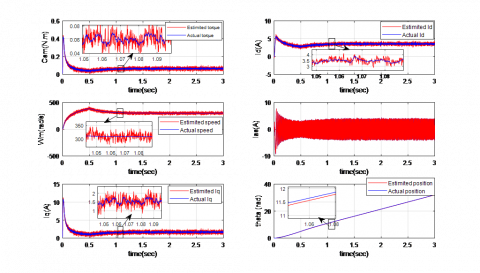Figure 6. State estimation result using trial and error method (Best MSE=0.0882)

Table 1. EKF performance using PSO algorithm

 Iterations Diagonal elements of matrices Q and R MSE qid qiq qω qθ rid riq 5 0.0105 0.0042 0.0484 0.1165 0.2145 0.0750 0.0225 10 0.0108 0.0024 0.0803 0.1154 0.1961 0.0755 0.0209 15 0.0014 0.0043 0.0898 0.0881 0.2245 0.1270 0.0171 20 0.0022 0.0035 0.1675 0.2367 0.2937 0.1569 0.0148

Table 2. EKF performances using GAs algorithm

 Generations Diagonal elements of matrices Q and R MSE qid qiq qω qθ rid riq 5 0.036 0.112 0.559 0.971 0.884 0.512 0.0317 10 0.014 0.104 0.856 1.047 0.942 0.655 0.0265 15 0.013 0.141 0.863 1.144 1.760 1.601 0.0216 20 0.013 0.014 0.818 1.258 0.650 0.347 0.0155

Table 3. EKF performances using BBO algorithm

 Iterations Diagonal elements of matrices Q and R MSE qid qiq qω qθ rid riq 5 0.1090 0.3750 8.8364 9.0673 0.1818 0.0838 0.0188 10 0.0881 0.4852 17.7553 12.3701 12.2714 4.1770 0.0178 15 0.5789 0.1584 6.1838 19.4921 17.0806 12.9395 0.0160 20 0.0127 0.0108 0.6934 0.5012 1.5007 1.5733 0.0138

Figure 7 shows that EKF tuned by PSO is able to generate accurate speed and position estimation and all the state variables (electromagnetic torque and currents) compared to conventional EKF regulates manually. The MSE reduced to 0.0148 after 20 iterations.

Table 1 illustrates Q and R optimized parameters with their equivalent MSEs acquired by PSO-EKF scheme presented in reference .

Table 2 presents GA-EKF process convergence, where MSE is reduced to 0.0155 after 20 iterations. GA parameters of are taken from reference  with the following data.

Initial population size 100; Probability of crossover 0.8; Mutation probability 0.01; Initial range of real-valued strings [0, 0.1]. Simulation investigations show that these proposed approaches give excellent estimations within 20 iterations compared to trial and error way. In Table 2, are presented the optimized parameters Q and R with their corresponding MSEs obtained by GA-EKF approach.

## 7.png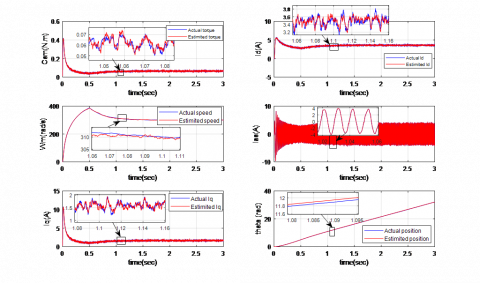Figure 7. State estimation result using PSO-EKF method (After 20 iterations, under load Cr=0.05 at t=0.5s)

## 8.png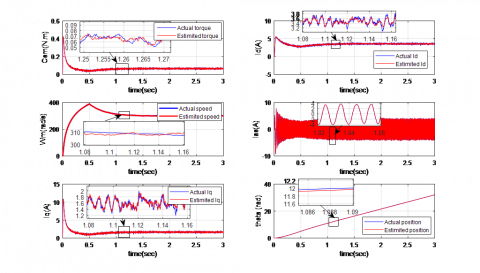Figure 8. Simulation results obtained by BBO-EKF (after 20 iterations, under load Cr=0.05 at t=0.5s)

## 9.png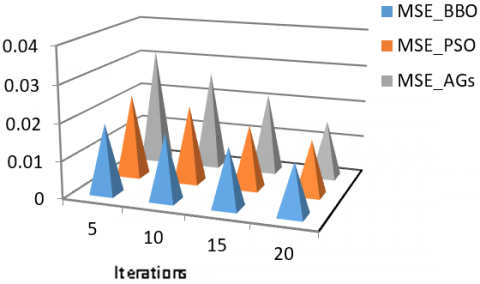Figure 9. Evolution of MSE versus EAs methods and iterations number

## 10a.png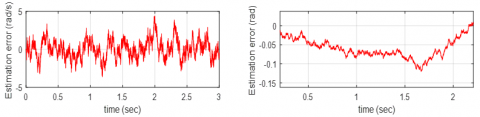(a) Speed and position estimation errors using EKF-AGs

## 10b.png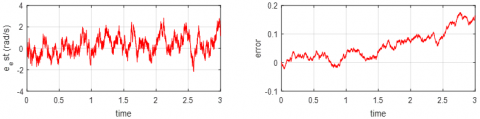(b) Speed and position estimation errors using EKF-PSO

## 10c.png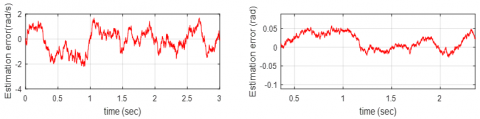(c) Speed and position estimation errors using EKF-BBO

Figure 10. Estimation errors of PMSM drive, using EKF tuned by different optimization techniques

Figure 8 shows the plotted simulation results representing the best optimal values of EKF parameters (20 iterations) employing BBO algorithm. It can be noted from this figure a perfect concordance between actual and estimated rotor speed and position in both transient and steady states. It can also be observed that speed and position estimation errors stay both within admissible limits.

Table 3 shows the convergence of BBO-EKF process for various numbers of iterations, where the best solution is a habitat with low MSE. The latter decreased to 0.0138 after 20 iterations. The BBO algorithm is running with parameters selected as follows: Population size (number of habitat) 20; Number of decision variables (SIVs):6; Immigration rate (I) and emigration rate (E): l; absorption coefficient α: 0.9 and probability mutation mmax: 0.1.

The proposed BBO-EKF is compared to trial-error method, GAs and PSO techniques. Note that EKF optimized by both GAs and PSO method a precise rotor speed and position can be achieved regarding the trial and error technique as illustrated in Tables 1, 2 and 3.

It is worth to note that the BBO-EKF technique has higher performances compared to EKF optimized by GAs or PSO methods in precision, MSE with BBO is less than the MSE with GA or PSO. In convergence rate BBO algorithm is faster than others, which proves the advantage of BBO-EKF method as illustrated in Figure 9, where it is gives the recapitulative of the evolution of MSE versus the three EAs methods treated above and iterations number.

In Figure 10, a comparison between the estimation errors obtained using EKF tuned by the three preceding optimization algorithms shows that the performance of the PMSM drive joint to BBO-EKF was enhanced than the PSO-EKF or AGs-EKF techniques.

Figure 11 presents the corresponding evolution of the fitness function MSE for the best solutions with respect to the number of iterations (case of 20 iterations) obtained through PSO-EKF and BBO-EKF algorithms.

## 11a.png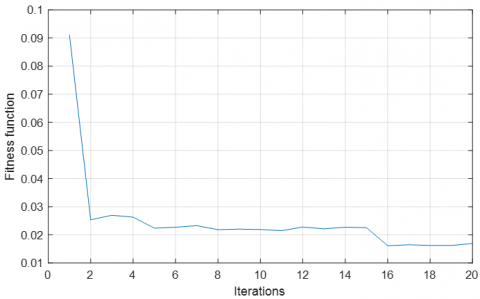a) Fitness function relative to PSO-EKF

## 11b.png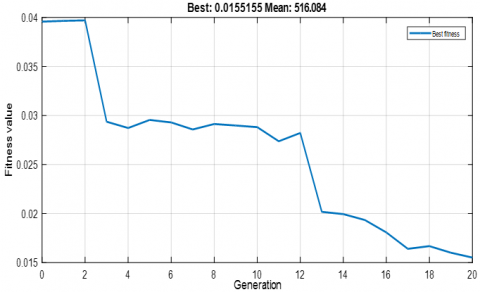b) Fitness function relative to AGs-EKF

## 11c.pngc) Fitness function relative to BBO-EKF

Figure 11. Evolution of the fitness function (MSE)

Evaluating the effectiveness of the proposed approach, the estimation based BBO-EKF are tested under load and reversal of the rotor speed. Figure12, shows the actual and estimated rotor speed and position of the PMSM drive under 0.05 N.m load with reversal speed at t=0.5s. Analyzing the results, it can be noted that the state estimation has stayed inside a very narrow error band, which encourages the employment of the developed estimation method for PMSM sensorless control. As shown in Figure12, the values of rotor speed and position estimated by BBO-EKF is very close to the actual values and offer precise estimation at low speeds when exposed to frequent reversals of speed.

## 12.png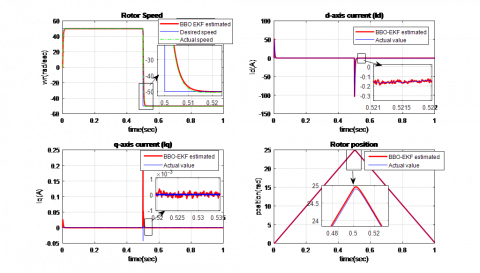Figure 12. Simulation results of PMSM drive based on BBO-EKF observer under load and speed variation

5. Conclusions

A combined BBO-EKF algorithm is proposed in this work as a technique to optimize the noise covariance matrices Q and R on which the EKF performance strongly depends.

A comparison between BBO-EKF with both approaches (PSO-EKF and AGs-EKF) was performed in the presence noises. The simulation results have confirmed that the estimation performance of PMSM drive with combined BBO-EKF technique is more efficiency compared to both optimization methods PSO-EKF and AGs-EKF. In terms of accuracy, after 20 iterations, the mean squared error (MSE) of the BBO-EKF approach is found to be 0.0138 however for both AGs-EKF and PSO-EKF algorithms are equal to 0.0148 and 0.0155 respectively. In convergence rate BBO algorithm is faster than others, which proves the advantage of BBO-EKF.

The estimation algorithm was also experimented under various load torque and speed variations. The results have showed high performances, encouraging the employment of the proposed technique for PMSM drives sensorless control.

Acknowledgment

The authors gratefully acknowledge the Algerian General Direction of Research (DGRSDT) for providing the facilities and the financial funding of this project.

Nomenclature
 ${{i}_{ds}},\,{{i}_{qs}}$ Stator currents in d-q coordinate system ${{v}_{ds}},\,{{v}_{qs}}$ Stator voltages in d-q coordinate system ${{\phi }_{m}}$ Rotor Magnetic flux ${{R}_{s}}$ Stator resistance $J$ Moment inertia of the rotor ${{f}_{r}}$ Vicous friction coefficient $p$ Pole pairs Number ${{L}_{d}}\text{ (}{{\text{L}}_{\text{q}}})$ Stator inductance in d-q coordinate system ${{\omega }_{r}}$ Rotor speed ${{\theta }_{r}}$ Rotor angle position ${{C}_{r}}$ Load torque
Appendix

PMSM Parameters used in Simulation

 Components Rating values Pn Vn Rs Ld Lq $\Phi$ J fr p Cn Rated power Rated voltage Stator resistance Direct axis inductance of stator Quadrature axis inductance of stator PM flux linkage Inertia of rotor Frictional coefficient Number of pole pairs Nominal torque 100 W 28 v 3.4 Ω 0.0121 H 0.0121 H 0.013 Wb 5.9e-5 kg.m2 10-4 N.m/rad/s 2 0.05 N.m
References

 Xu, D., Wang, B., Zhang, G., Wang, G., Yu, Y. (2018). A review of sensorless control methods for AC motor drives. CES Transactions on Electrical Machines and Systems, 2(1): 104-115. https://doi.org/10.23919/TEMS.2018.8326456

 Vas, P. (1998). Sensorless, Vector and Direct Torque Control. Oxford University Press, London.

 Ramana, P., Mary, K.A., Kalavathi, M.S., Swathi, A. (2015). Parameter estimation of permanent magnet synchronous motor a review. I-Manager's Journal on Electrical Engineering, 9(2): 49-59. https://doi.org/10.1109/EPETSG.2015.7510176

 Akin, B., Orguner, U., Ersak, A., Ehsani, M. (2006) Simple derivative-free nonlinear state observer for sensorless AC drives. IEEE/ASME Transactions on Mechatronics, 11(5): 634-642. https://doi.org/10.1109/TMECH.2006.882996

 Yin, Z., Gao, F., Zhang, Y., Du, C., Li, G., Sun, X. (2019). A review of nonlinear Kalman filter appling to sensorless control for AC motor drives. CES Transactions on Electrical Machines and Systems, 3(4): 351-362. https://doi.org/10.30941/CESTEMS.2019.00047

 Shanmao, G., Fengyou, H., Guojun, T., Shengwen, Y. (2009). A review of sensorless control technology of permanent magnet synchronous motor. Transactions of China Electrotechnical Society, 24(11): 14-20. https://doi.org/10.1155/2016/3916231

 Ananthasayanam, M.R., Mohan, M.S., Naik, N., Gemson, R.M.O. (2016). A heuristic reference recursive recipe for adaptively tuning the Kalman filter statistics part-1: formulation and simulation studies. Sādhanā, 41(12): 1473-1490. https://doi.org/10.1007/s12046-016-0562-z

 Ahn, H., Park, H., Kim, C., Lee, H. (2020). A review of state-of-the-art techniques for PMSM parameter identification. Journal of Electrical Engineering & Technology, 15(3): 1177-1187. https://doi.org/10.1007/s42835-020-00398-6

 Luenberger, D.G. (1971). An introduction to observers. IEEE Transactions on Automatic Control, 16(6): 596-602, https://doi.org/10.1109/TAC.1971.1099826

 Kalman, R.E. (1960). A new approach to linear filtering and prediction problems. Transactions of the ASME Journal of Basic Engineering, 82(1): 35-45. https://doi.org/10.1115/1.3662552

 Tety, P., Konaté, A., Asseu, O., Soro, E., Yoboué, P., Kouadjo, A.R. (2015). A robust extended Kalman filter for speed-sensorless control of a linearized and decoupled PMSM drive. Engineering, 7(10): 691-699. http://dx.doi.org/10.4236/eng.2015.710060

 Sriprang, S., Nahid-Mobarakeh, B., Pierfederici, S., Takorabet, N., Bizon, N., Kumam, P., Mungporn, P., Thounthong, P. (2018). Robust flatness control with extended Luenberger observer for PMSM drive. In 2018 IEEE Transportation Electrification Conference and Expo, Asia-Pacific (ITEC Asia-Pacific), pp. 1-8. https://doi.org/10.1109/ITEC-AP.2018.8432600

 Ye, S. (2019). Design and performance analysis of an iterative flux sliding-mode observer for the sensorless control of PMSM drives. ISA Transactions, 94(11): 255-264. https://doi.org/10.1016/j.isatra.2019.04.009

 Wu, K., Sun, C. (2019). Sensorless speed control of PMSM via extended state observer. Chinese Control Conference (CCC), Guangzhou, China, pp. 508-512. https://doi.org/10.23919/ChiCC.2019.8865805

 Gopinath, G.R., Das, S.P. (2018). An extended Kalman filter based sensorless permanent magnet synchronous motor drive with improved dynamic performance. IEEE International Conference on Power Electronics, Drives and Energy Systems (PEDES), Chennai, India, pp. 1-6. https://doi.org/10.1109/PEDES.2018.8707900

 Pal, A., Das, S., Chattopadhyay, A.K. (2018). An improved rotor flux space vector based MRAS for field-oriented control of induction motor drives. IEEE Transactions on Power Electronics, 33(6): 5131-5141. https://doi.org/10.1109/TPEL.2017.2657648

 Adamu, S.S., Lawan, M.B. (2017). Permanent magnet synchronous motor rotor position estimation using fuzzy-based sliding mode observer. 9th IEEE-GCC Conference and Exhibition (GCCCE), Manama, Bahrain, pp. 1-5. https://doi.org/10.1109/IEEEGCC.2017.8448219

 Feifei, H., Zhonghua, W., Yueyang, L., Tongyi, H. (2015). Sensorless speed control of permanent magnet synchronous motor based on RBF neural network. Proceedings of the 34th Chinese Control Conference (CCC '15) IEEE, Hangzhou, China, pp. 4325-4330. https://doi.org/10.23919/ChiCC.2018.8483974

 Gowda, M., Ali, W., Cofie, P., Fuller, J. (2013). Design and digital implementation of controller for PMSM using extended Kalman filter. Circuits and Systems, 4(8): 489-497. http://dx.doi.org/10.4236/cs.2013.48064

 Medjghou, A., Ghanai, M., Chafaa, K. (2018). Improved feedback linearization control based on PSO optimization of an extended Kalman filter. Optimal Control Applications and Methods, 39(6): 1-16. https://doi.org/10.1002/oca.2454

 Shi, K.L., Chan, T.F., Wong, Y.K., Ho, S.L. (2002). Speed estimation of an induction motor drive using an optimized extended Kalman filter. in IEEE Transactions on Industrial Electronics, 49(1): 124-133. https://doi.org/10.1109/41.982256

 Laamari, Y., Chafaa, K., Athamena, B. (2015). Particle swarm optimization of an extended Kalman filter for speed and rotor flux estimation of an induction motor drive. Electrical Engineering, 97(2): 129-138. https://doi.org/10.1007/s00202-014-0322-1

 Kennedy, J., Eberhart, R.C. (1995). Particle swarm optimization. In Proceedings of the IEEE International Conference on Neural Networks, Piscataway, USA, 1942-1948. https://doi.org/10.1007/978-0-387-30164-8_630

 Simon, D. (2008). Biogeography-based optimization. IEEE Transactions on Evolutionary Computation, 12(6): 702-713. https://doi.org/10.1109/TEVC.2008.919004

 Kannan, R., Gayathri, N., Natarajan, M., Sankarkumar, R.S., Iyer, L.V., Kar, N.C. (2016). Selection of PI controller tuning parameters for speed control of PMSM using biogeography based optimization algorithm. 2016 IEEE International Conference on Power Electronics, Drives and Energy Systems (PEDES), Trivandrum, India, 1-6. https://doi.org/10.1109/PEDES.2016.7914260

 Wang, J.S., Song, J.D. (2016). Migration ratio model analysis of biogeography-based optimization algorithm and performance comparison. International Journal of Computational and Intelligence Systems, 9(3): 544-558. https://doi.org/10.1080/18756891.2016.1175817

 Ma, H., Simon, D., Siarry, P., Yang, Z., Fei, M. (2017). Biogeography-based optimization: A 10-year review. IEEE Transactions on Emerging Topics in Computational Intelligence, 1(5): 391-407. https://doi.org/10.1109/TETCI.2017.2739124